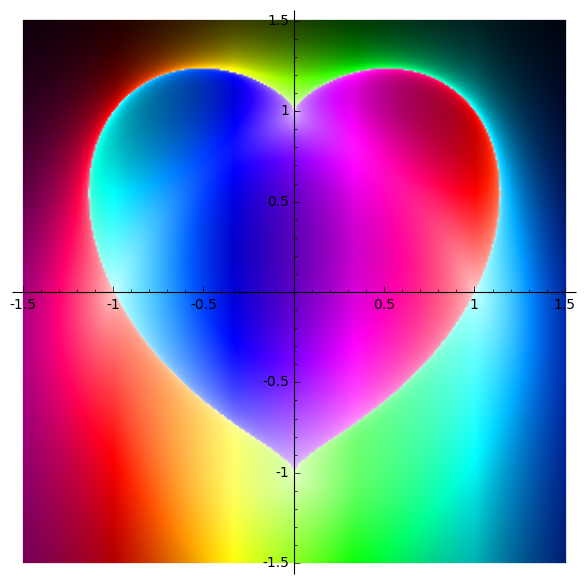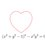# The Beauty of MathThis Post is an attempt to collect beautiful mathematical art including but not limited to plots, complex plots, contour plots, fractals, surfaces and tilings.

In the comments, post your favorites. A description of what you post would be much appreciated.

By the way, read this post for the code of the attached heart and a few other plots.

By the way, my avatar is also one of my favorites.7 years, 1 month ago

This discussion board is a place to discuss our Daily Challenges and the math and science related to those challenges. Explanations are more than just a solution — they should explain the steps and thinking strategies that you used to obtain the solution. Comments should further the discussion of math and science.

When posting on Brilliant:

• Use the emojis to react to an explanation, whether you're congratulating a job well done , or just really confused .
• Ask specific questions about the challenge or the steps in somebody's explanation. Well-posed questions can add a lot to the discussion, but posting "I don't understand!" doesn't help anyone.
• Try to contribute something new to the discussion, whether it is an extension, generalization or other idea related to the challenge.

MarkdownAppears as
*italics* or _italics_ italics
**bold** or __bold__ bold
- bulleted- list
• bulleted
• list
1. numbered2. list
1. numbered
2. list
Note: you must add a full line of space before and after lists for them to show up correctly
paragraph 1paragraph 2

paragraph 1

paragraph 2

[example link](https://brilliant.org)example link
> This is a quote
This is a quote
    # I indented these lines
# 4 spaces, and now they show
# up as a code block.

print "hello world"
# I indented these lines
# 4 spaces, and now they show
# up as a code block.

print "hello world"
MathAppears as
Remember to wrap math in $$ ... $$ or $ ... $ to ensure proper formatting.
2 \times 3 $2 \times 3$
2^{34} $2^{34}$
a_{i-1} $a_{i-1}$
\frac{2}{3} $\frac{2}{3}$
\sqrt{2} $\sqrt{2}$
\sum_{i=1}^3 $\sum_{i=1}^3$
\sin \theta $\sin \theta$
\boxed{123} $\boxed{123}$

Sort by:

The heart curve can simply be written in polar coordinate See here

Plot this parametric equation in $t$ as $0

$y_{1}=2+2\sin \ t$ $x_{1}=2+2\cos \ t$

$y_{2}=3+0.1\sin \ t$ $x_{2}=1+0.1\cos \ t$

$y_{3}=3+0.1 \sin \ t$ $x_{3}=3+0.1 \cos \ t$

$y_{4}=2-\sin \ 0.5t$ $x_{4}=2+ \cos \ 0.5t$

It will bring a smile:)))

- 7 years, 1 month ago

Thanks cool!

- 7 years, 1 month agosssss

- 6 years, 9 months ago

Wolfram Blog has an article on converting any image to a formula.https://fbcdn-sphotos-a-a.akamaihd.net/hphotos-ak-xaf1/v/t1.0-9/s526x395/106651045876852413364147639235888110981929n.jpg?oh=52bc7675305c2fe4a3e1090f3f04476e&oe=54C41262&gda=14213948218b7d65673af22a9fef39d6d88367d0b7

- 6 years, 9 months ago

Oh, let me offer this one that I made up: Knot Or Not?. Complicated parametric equations plot this one out, but the fun problem is, "If you tried to untangle this, will it just end up in a knot, or can it be untangled into a simple loop?"

- 7 years, 1 month ago

It can be untangled into the trivial knot, ie, the loop. Yes?

- 7 years, 1 month ago

Like how the CIA tweeted today, I can neither confirm nor deny this!

- 7 years, 1 month ago

I'll have to get some long pieces of strings and try to make this knot and see for myself.

- 7 years, 1 month ago

Enter this into google: sqrt(cos(x))cos(300x)+sqrt(abs(x))-0.7)(4-x*x)^0.01 sqrt(6-x^2) from-4.5 to 4.5

- 7 years, 1 month ago